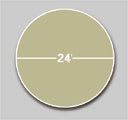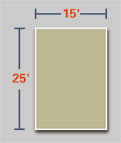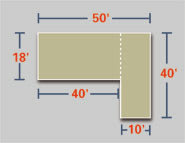Open Monday — Friday: 7:00 AM - 6:00 PM,
Saturday: 8:00 AM - 3:00 PM

# Estimating Resources

Percent Passing Range

## Calculating Circles

How to calculate Linear Feet Around a CircleFormula: Diameter x 3.1416 = Linear Feet Around a Circle

Example: Length Around an 18 foot diameter round Swimming Pool

18 ft x 3.1416 = 56.55 ft (total linear feet around pool)

To add 2 feet of stone (3 inches deep) around this pool: 56.55 feet x 2 feet= 113.10 feet

113.10 feet x 0.25 (3 inch depth) = 28.28 Cubic Feet

28.28 / 27 = 1.05 Cubic Yards

How to calculate the square footage inside a circleFormula: Length x Width x 0.7854 = Square Feet

Example: Square footage inside a 24 foot diameter round Swimming Pool

24 feet x 24 feet = 576 feet

576 feet x 0.7854 = 452.39 Square Feet (total inside pool)

To add 4 inches of sand evenly across pool:
452.39 feet x .33 (4 inches depth) = 149.29 Cubic Feet

149.29 / 27 = 5.53 Cubic Yards

To be safe, always round up. You would order 6 Cubic Yards of sand.

How to calculate coverage for a standard driveway or padFormula: Diameter x 3.1416 = Linear Feet Around a Circle

Example: Length Around an 18 foot diameter round Swimming Pool

18 ft x 3.1416 = 56.55 ft (total linear feet around pool)

To add 2 feet of stone (3 inches deep) around this pool: 56.55 feet x 2 feet= 113.10 feet

113.10 feet x 0.25 (3 inch depth) = 28.28 Cubic Feet

28.28 / 27 = 1.05 Cubic Yards

How to calculate coverage for an L-shaped driveway or padExample: L-Shaped Driveway or Pad to be covered with stone, 7 inches deep. The calculation is done in two sections.

18 feet x 40 feet = 720 Square Feet

10 feet x 40 feet = 400 Square Feet

400 feet + 720 feet = 1,120 Square Feet

1,120 x .58 (7 inch Depth) = 649.60 Cubic Feet

649.60 / 27 = 24.06 Cubic Yards

## Depth Estimating

Depth Chart

• 1 inch = 0.08 feet
• 2 inches = 0.17 feet
• 3 inches = 0.25 feet
• 4 inches = 0.33 feet
• 5 inches = 0.42 feet
• 6 inches = 0.50 feet
• 7 inches = 0.58 feet
• 8 inches = 0.67 feet
• 9 inches = 0.75 feet
• 10 inches = 0.83 feet
• 11 inches = 0.92 feet
• 12 inches = 1.00 feet
• 13 inches = 1.08 feet
• 14 inches = 1.17 feet
• 15 inches = 1.25 feet
• 16 inches = 1.33 feet
• 17 inches = 1.42 feet
• 18 inches = 1.50 feet

General Quantity Formulas

Length x Width = Square Feet

Square Feet x Depth = Cubic Feet

Cubic Feet / 27 = Cubic Yards

Important

When figuring quantities, remember to: